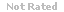Loading... Please wait...

## A numerical value used as a summary measure for a sample, such as sample mean, is known as a population parameter?

Price:
\$12.99

### Product Description

A numerical value used as a summary measure for a sample, such as sample mean, is known as a population parameter?

QUESTION 2

1. The 50th percentile is the

QUESTION 3

1. The difference between the largest and the smallest data values is the

QUESTION 4

1. When data are positively skewed, the mean will usually be

QUESTION 5

1. The numerical value of the standard deviation can never be

QUESTION 6

1. Which of the following symbols represents the standard deviation of the population?

QUESTION 7

1. Which of the following symbols represents the mean of the population?

QUESTION 8

1. Which of the following symbols represents the mean of the sample?

QUESTION 9

1. Two events are mutually exclusive

QUESTION 10

1. The range of probability is

QUESTION 11

1. The sum of the probabilities of two complementary events is

QUESTION 12

1. The union of events A and B is the event containing

QUESTION 13

1. Events A and B are mutually exclusive. Which of the following statements is also true?

QUESTION 14

1. The set of all possible sample points (experimental outcomes) is called

QUESTION 15

1. If A and B are independent events, then

### Customers Who Viewed This Product Also Viewed

• \$16.99
• \$22.99
• \$8.99
• \$8.99
• \$19.99

• \$12.99